## Cell Highlights¶

• FormulaChop looks for certain functions and highlights the cells in their precedent ranges that contribute to their results.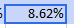A dark outline shows highlighted cells

Tip

Any time a cell is highlighted, the label for its range becomes a link to only the highlighted cells

This can be very useful to focus on only the cells that are relevant to a calculation

Example

Navigate to the ‘Examples’ tab of `this file` to try the examples below for yourself

### INDEX()¶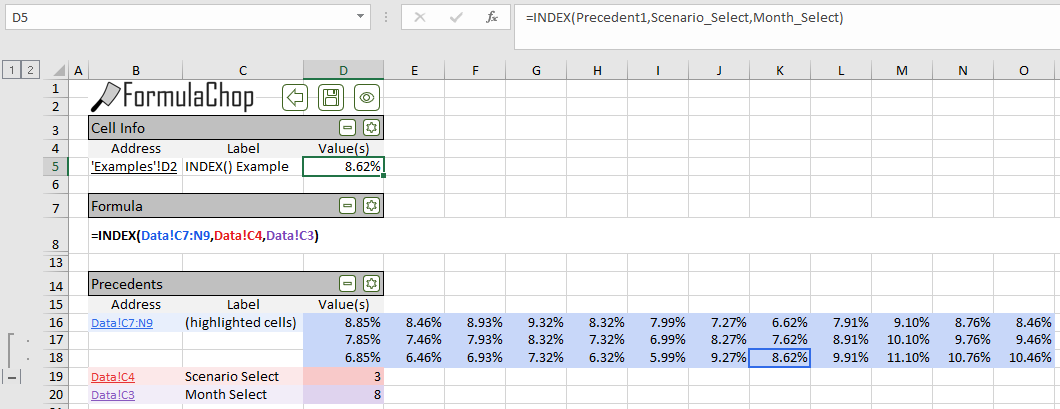FormulaChop highlights the cell(s) returned by the `INDEX()` function

### LOOKUP()¶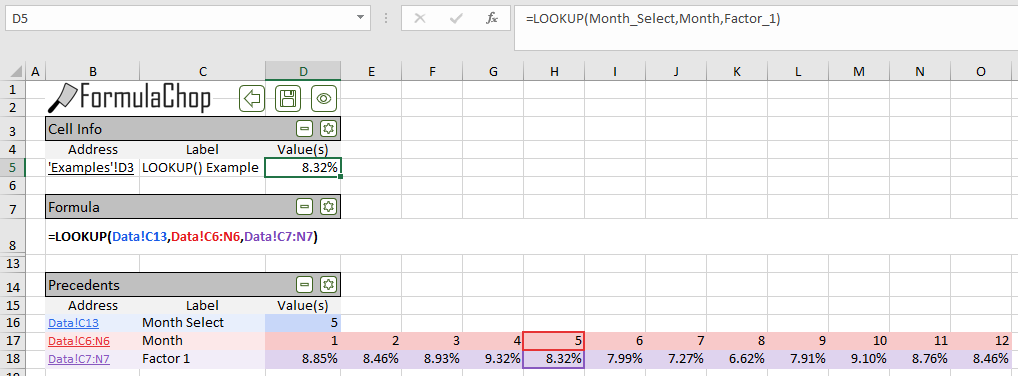FormulaChop highlights the cell(s) in which the search value is found, as well as the return value.

• This works for `LOOKUP()`, `HLOOKUP()` and `VLOOKUP()`

### CHOOSE()¶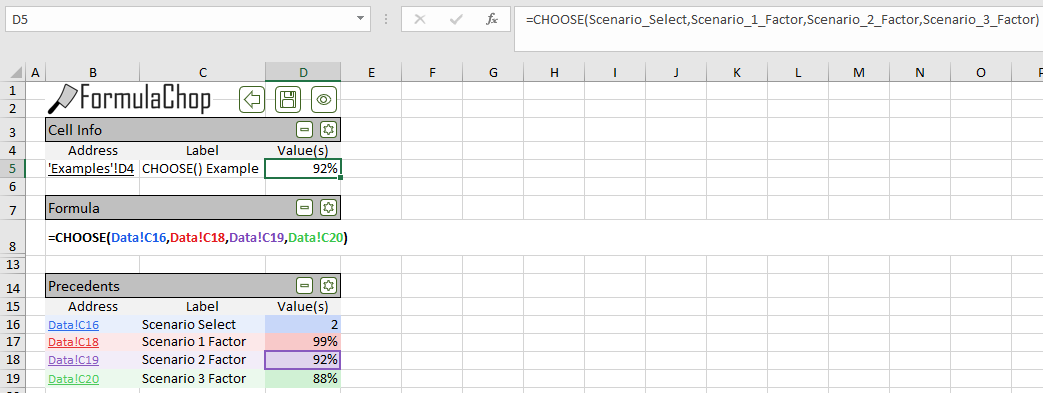FormulaChop highlights cell(s) returned by `CHOOSE()`

### SUMIF(), COUNTIF(), AVERAGEIF()¶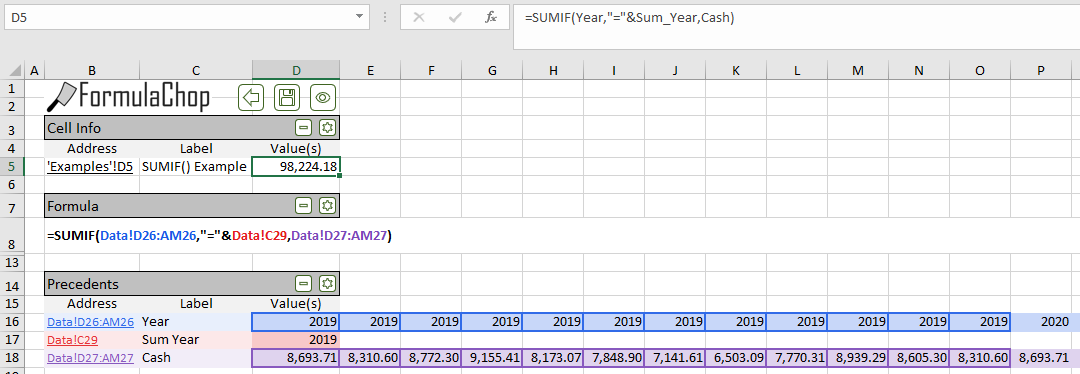FormulaChop highlights the cell(s) where the condition is true, as well as the cell(s) being summed/counted/averaged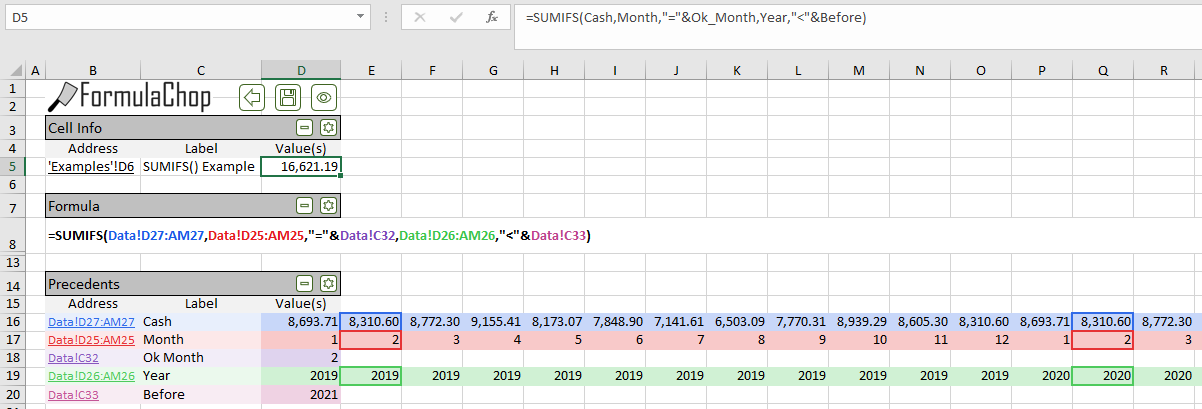This works for the plural versions of these functions: `SUMIFS()` and `COUNITFS()`

### MATCH()¶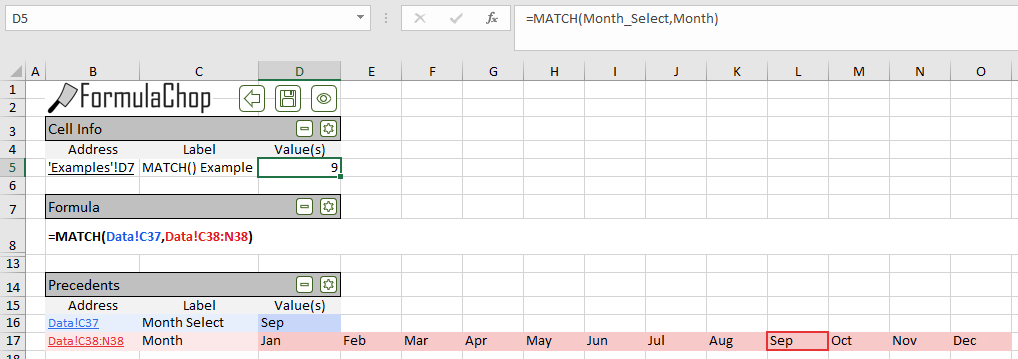FormulaChop highlights matching cell(s)

• FormulaChop supports advanced formula constructions which are used in large, complex spreadsheets.

Example

See `this file` to try the examples below for yourself

### Formulas Which Return Ranges¶

• Formulas can be written such that they return a range, instead of a single value.
• In this situation, Excel will pick the value in the returned range that aligns with the calling cell.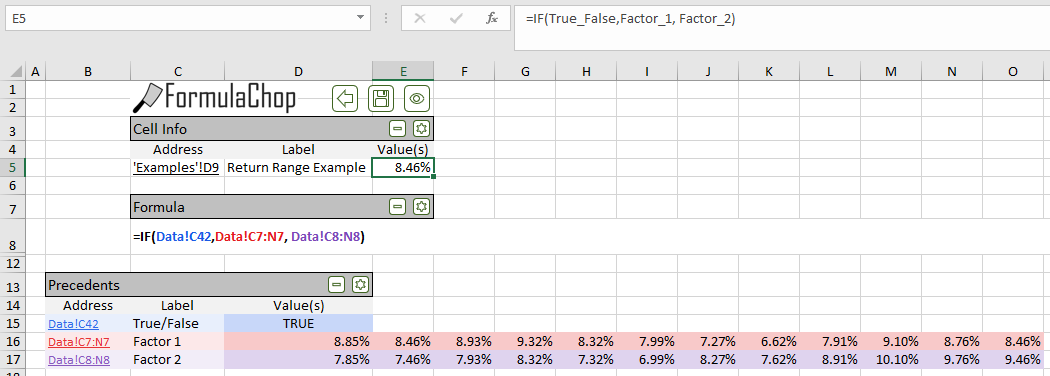FormulaChop handling a formula which returns a range

Example:

The figure above shows FormulaChop output from cell `D9`. The chopped formula is:

`=IF(True_False,Factor_1, Factor_2)`

This `IF()` statement returns a range of cells, depending on the value in `True_False`.

Excel uses the relative alignment of the calling cell, `G3` and the returned range, `Factor_1` (`F7:Q7`), to decide which value to in the returned range to show. Because the calling cell is in column `G`, the value in column `G` of the range `Factor_1` will be returned

FormulaChop recognizes this situation and recreates all the ranges’ alignment on the FormulaChop Tab.

• A common example of this situation is the use of `INDEX()` with a `0` argument.
• When `0` is given as an argument for a row or column, `INDEX()` returns the entire row or column.
• Because `INDEX()` is returning a range, Excel will use the relative alignment of the calling cell and the resulting range to give the formula’s single result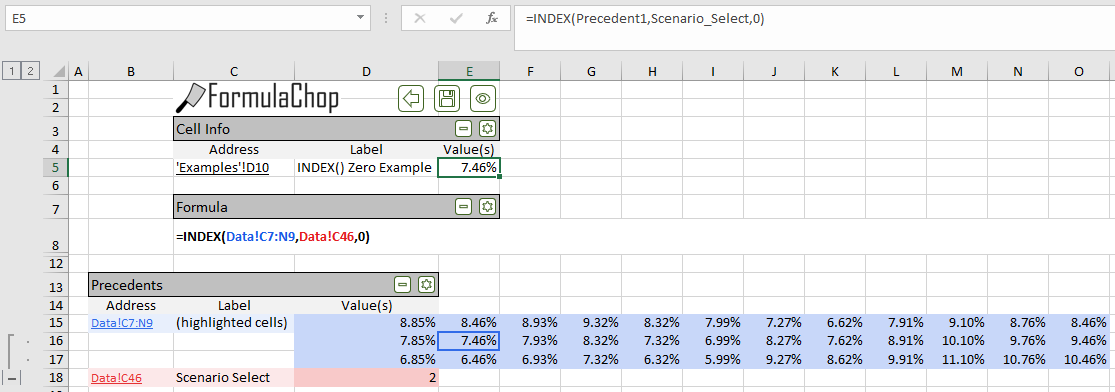FormulaChop handling INDEX() Zero Argument

Example

The figure above shows FormulaChop output from cell `D10`. The chopped formula is:

`=INDEX(Precedent1,Scenario_Select,0)`

Instead of specifying a column, this formula uses `0` as its column argument.

Because the cell containing this formula is in column `G`, `INDEX()` will use column `G` in the range `Precedent1` (`F6:Q8`). This feature also applies when the calling cell is on a different tab from the range in the first argument to `INDEX()`.

### OFFSET()¶

• Unique among all Excel functions, the `OFFSET()` function is able to depend on ranges without explicitly referring to them.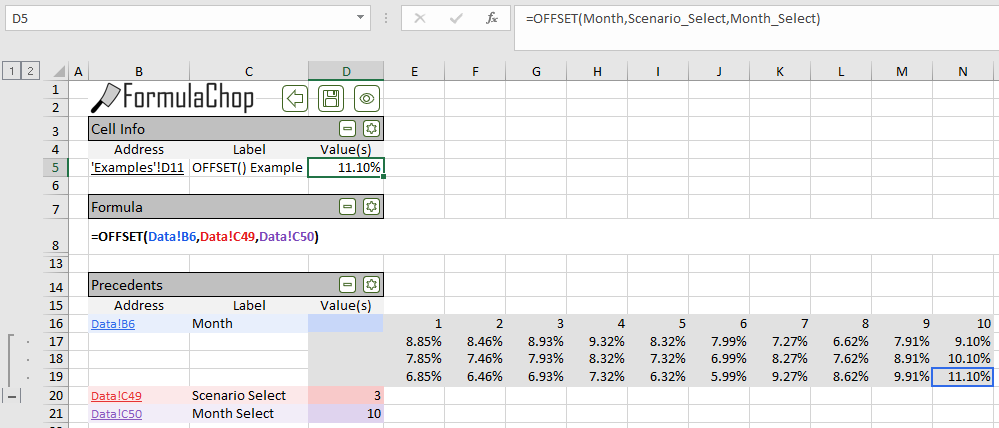FormulaChop handling an `OFFSET()` function

• FormulaChop evaluates each `OFFSET()` in the formula, and determines the range it is referring to, and copies that range to the FormulaChop Tab

### INDIRECT()¶

• The `INDIRECT()` function accepts as text the address of a range in the workbook, then it returns that range.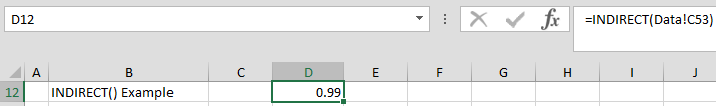A formula using the `INDIRECT()` function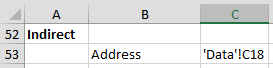The cell referenced by the `INDIRECT()` function: `Data!C50`. Notice it contains a cell address as text.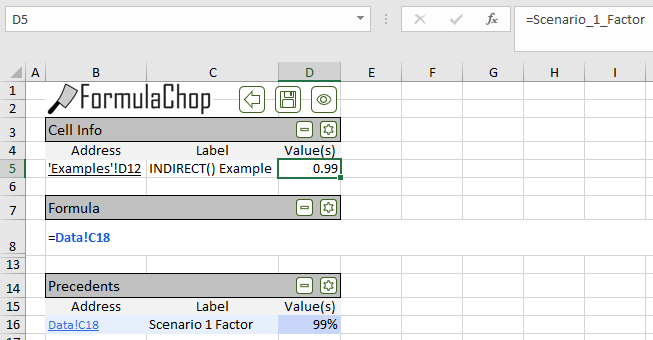FormulaChop handling an `INDIRECT()` function. The `INDIRECT()` function has been replaced by its result: `Data!C18`.

• FormulaChop evaluates each call to `INDIRECT()` and finds its resulting range
• Then FormulaChop replaces the call to `INDIRECT()` in the formula with the range
• The range returned by `INDIRECT()` appears on the FormulaChop Tab

### Dynamic Ranges¶

• Excel allows two functions, `OFFSET()` and `INDEX()`, to be used in the place of a cell when defining a range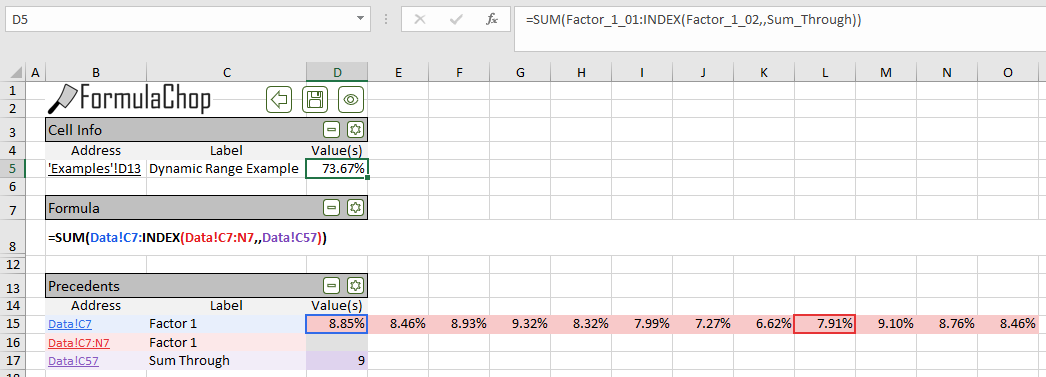FormulaChop handling a Dynamic Range.

Example

The figure above shows FormulaChop output from cell `D12`. The chopped formula is:

`=SUM(Factor_1_01:INDEX(Factor_1_02,,Sum_Through))`

• The value of `Sum_Through` (`F11`) is `9`

• `Factor_1_01` is the range `B10`, `Factor_1_02` is the range `B10:M10`

• `INDEX(Factor_1_02,,Sum_Through)` will return the cell `J10`

• `B10` + `9` columns = `J10`
• Now, the formula reads `SUM(B10:J10)`

The value in `Sum_Through` defines the size of the range being summed. This is what makes the range “dynamic”

• FormulaChop is able to handle Dynamic Ranges by only showing a single range on the FormulaChop Tab
• The start and end of the Dynamic Range is highlighted

### 3D Ranges¶

• Excel allows ranges to be defined across many tabs. This is called a 3D Range.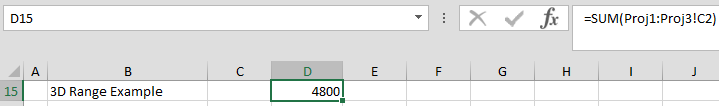A formula with a 3D range. Notice the range of tabs specified: `Proj1:Proj3`.FormulaChop handling a 3D Range. Notice the 3D range has been replaced with a list of ranges.

Example

The figure above shows FormulaChop output from a cell using a 3D Range. This workbook has tabs `Proj1`, `Proj2` and `Proj3`. Each of these tabs has the same layout, with a “Capacity” value in cell `C2`. The chopped formula is:

`=SUM('Proj1:Proj3'!C2)`

This formula sums the values in cell `C2` in all of the tabs between `Proj1` and `Proj3`.

• FormulaChop looks for 3D ranges in the formula, and replaces them with a comma-separated list of ranges on each tab

## Labels¶

• FormulaChop does its best to automatically find the labels for the ranges in the Precedents and Dependents sections

• FormulaChop will look for labels

• Horizontally if the range is a row (determined by the Orientation setting)
• Vertically if the range is a column (determined by the Orientation setting)
• In the direction set by the Orientation setting if the range is a single cell. Then in the other direction if no label is found.
• The start and direction of the search depends on the setting of Label Search Direction

• Out<-Inside The search starts from the first cell in the precedent and steps outward
• Outside->In The search starts from the first row/column of the worksheet and steps inward
• If FormulaChop finds a cell containing text, and the text meets some criteria, FormulaChop decides that the text is the label

• Ranges that are blocks (i.e. have more than 2 rows and columns) are only labelled if they are named ranges

### Label Criteria¶

While FormulaChop is looking for a label, it will skip a cell if:

• Cell does not contain text
• Cell is empty or blank
• Text is fewer than 3 characters long
• Text is surrounded by parentheses or brackets (e.g. “(kWh)” and “[kWh]” would not be considered labels)
• Text is “USD”

### Custom Criteria¶

You may specify your own text for FormulaChop to ignore while searching for labels

FormulaChop will look for a Named Range named `fcIgnoreText`

• Any text inside `fcIgnoreText` will be ignored when FormulaChop searches for labels
• This range may be any shape or size, and may be on any tab
• Locating `fcIgnoreText` on the FormulaChop Tab is not recommended

### Rules for Turning Labels into Range Names¶

FormulaChop follows these rules when labels are being used to name precedent ranges

• Characters which are invalid in Named Ranges will be replaced with an underscore (“_”)

Example

A range with the label “Special Expenses (\$)” would be named “Special_Expenses____”

A range with the label “11th” would be named “_1th”

• Leading underscores will be removed if possible

Example

A range with the label “(-) Cash Distributions” would be named “Cash_Distributions”

A range with the label “101st” would be named “_01st”

• Repeat names will be numbered

Example

Two ranges with the label “date” would be named “date_01” and “date_02”

• Labels which could be interpreted as cell addresses will not be labelled

Example

A range with the label “P50” will not be named, because “P50” is the address of a cell

• Ranges with no label are named “PrecedentX”, where X is position in the order of appearance in the formula

Example

A range with no label, which appears 3rd in the formula, would be named “Precedent3”

## Unsupported Functions¶

FormulaChop is unable to support some Excel functions. FormulaChop will not run on formulas which contain these functions.

• In general, this is because these functions rely on aspects of the precedents cells which cannot be copied to the FormulaChop Tab
• For example, the `ROW()` function returns the row number of its argument
• FormulaChop rebuilds the formula on the FormulaChop Tab, and would be unable to always place the precedent cells in the same row as the chopped formula

### Unsupported Function List¶

• `CELL()`
• `COLUMN()`
• `FILTER()`
• `FORMULATEXT()`
• `ROW()`
• `SINGLE()`
• `SORT()`
• `TRANSPOSE()`
• `UNIQUE()`

### Array Formulas¶

FormulaChop is unable to support Array Formulas.

• Because FormulaChop is designed to only be run on one formula in one cell, it is unable to copy an array of cells to the FormulaChop Tab

### `INDIRECT()` Array Output¶

FormulaChop is unable to support formulas which use an INDIRECT() function that returns an array of ranges. In this context, there is no way to replace the `INDIRECT()` function with an ordinary range.

## Deleting The FormulaChop Tab¶

• The FormulaChop Tab is automatically deleted before the spreadsheet is saved or closed
• Any tabs which were saved using the Save command (or renamed manually) will not be deleted
• Running FormulaChop will not require the spreadsheet to be saved unless:
• It needed to be saved before FormulaChop was run
• Calculating the spreadsheet (i.e. pressing `F9`) causes it to require saving

## FormulaChop History¶

• FormulaChop saves a history of cells which have been chopped
• To go back in the history, use `Ctrl + Shift + V` (see Chop Commands)
• To go forward in the history, use `Ctrl + Shift + F` (see Chop Commands)
• If you are back in the history and you chop a new cell, this cell will become the latest in the history